# How to Calculate and Solve for Elastic Modulus on Longitudinal Direction | Composites

The elastic modulus on longitudinal direction is illustrated by the image below.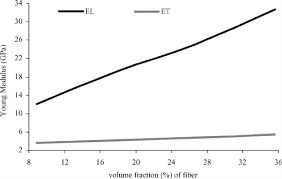To compute for elastic modulus on longitudinal direction, three essential parameters are needed and these parameters are Elastic Modulus of the Matrix (Em), Elastic Modulus of the Fibre (Ef) and Volume Fraction of the Fibre (Vf).

The formula for calculating elastic modulus on longitudinal direction:

Eu = Em(1 – Vf) + EfVf

Where:

Eu = Elastic Modulus on Longitudinal Direction
Em = Elastic Modulus of the Matrix
Ef = Elastic Modulus of the Fibre
Vf = Volume Fraction of the Fibre

Let’s solve an example;
Find the elastic modulus on longitudinal direction when the elastic modulus of the matrix is 12, the elastic modulus of the fibre is 10 and volume fraction of the fibre is 4.

This implies that;

Em = Elastic Modulus of the Matrix = 12
Ef = Elastic Modulus of the Fibre = 10
Vf = Volume Fraction of the Fibre = 4

Eu = Em(1 – Vf) + EfVf
Eu = (12)(1 – 4) + (10)(4)
Eu = (12)(-3) + (40)
Eu = (-36) + (40)
Eu = 4

Therefore, the elastic modulus on longitudinal direction is 4 Pa.

Nickzom Calculator – The Calculator Encyclopedia is capable of calculating the elastic modulus on longitudinal direction.

To get the answer and workings of the elastic modulus on longitudinal direction using the Nickzom Calculator – The Calculator Encyclopedia. First, you need to obtain the app.

You can get this app via any of these means:

You can also try the demo version via https://www.nickzom.org/calculator

Apple (Paid) – https://itunes.apple.com/us/app/nickzom-calculator/id1331162702?mt=8
Once, you have obtained the calculator encyclopedia app, proceed to the Calculator Map, then click on Materials and Metallurgical under Engineering.Now, Click on Composites under Materials and Metallurgical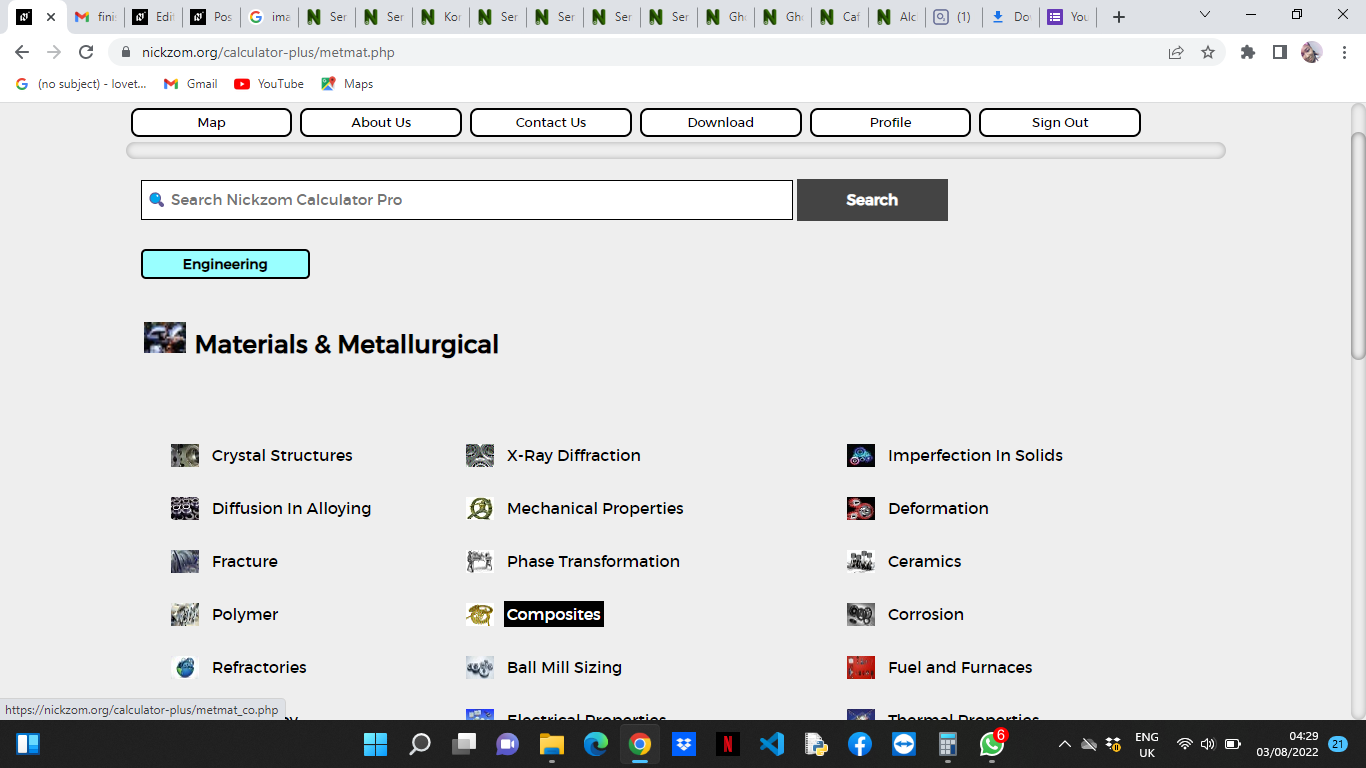Now, Click on Elastic Modulus on Longitudinal Direcction under Composites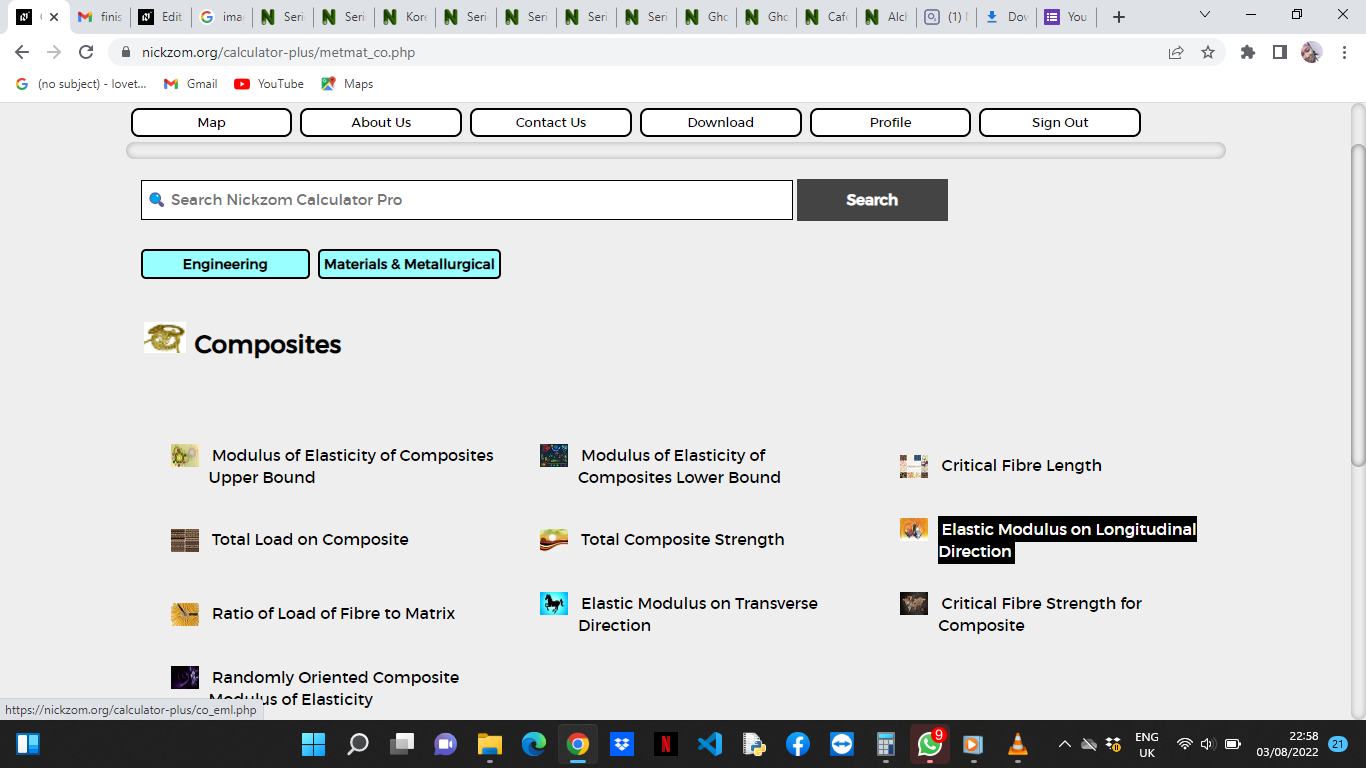The screenshot below displays the page or activity to enter your values, to get the answer for the elastic modulus on longitudinal across according to the respective parameter which is the Elastic Modulus of the Matrix (Em), Elastic Modulus of the Fibre (Ef) and Volume Fraction of the Fibre (Vf).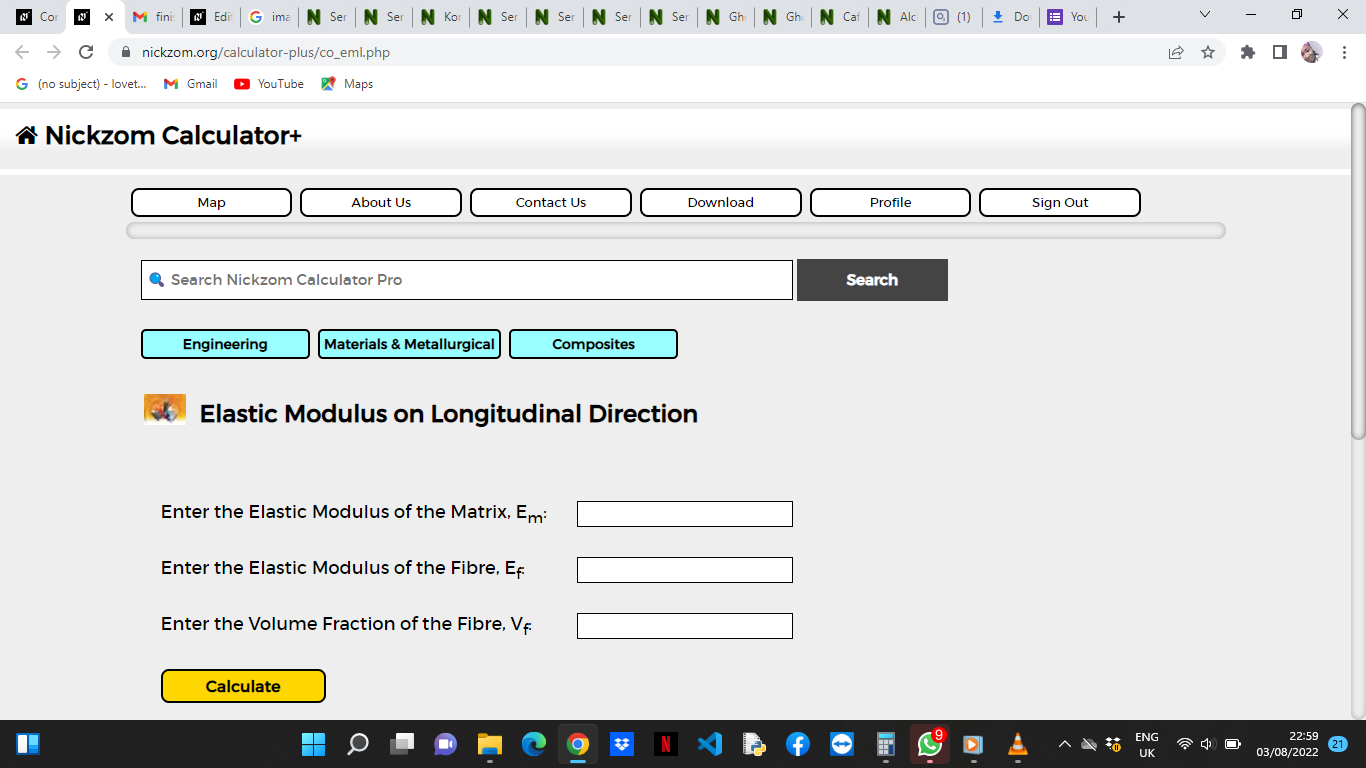Now, enter the values appropriately and accordingly for the parameters as required by the Elastic Modulus of the Matrix (Em) is 12, Elastic Modulus of the Fibre (Ef) is 10 and Volume Fraction of the Fibre (Vf) is 4.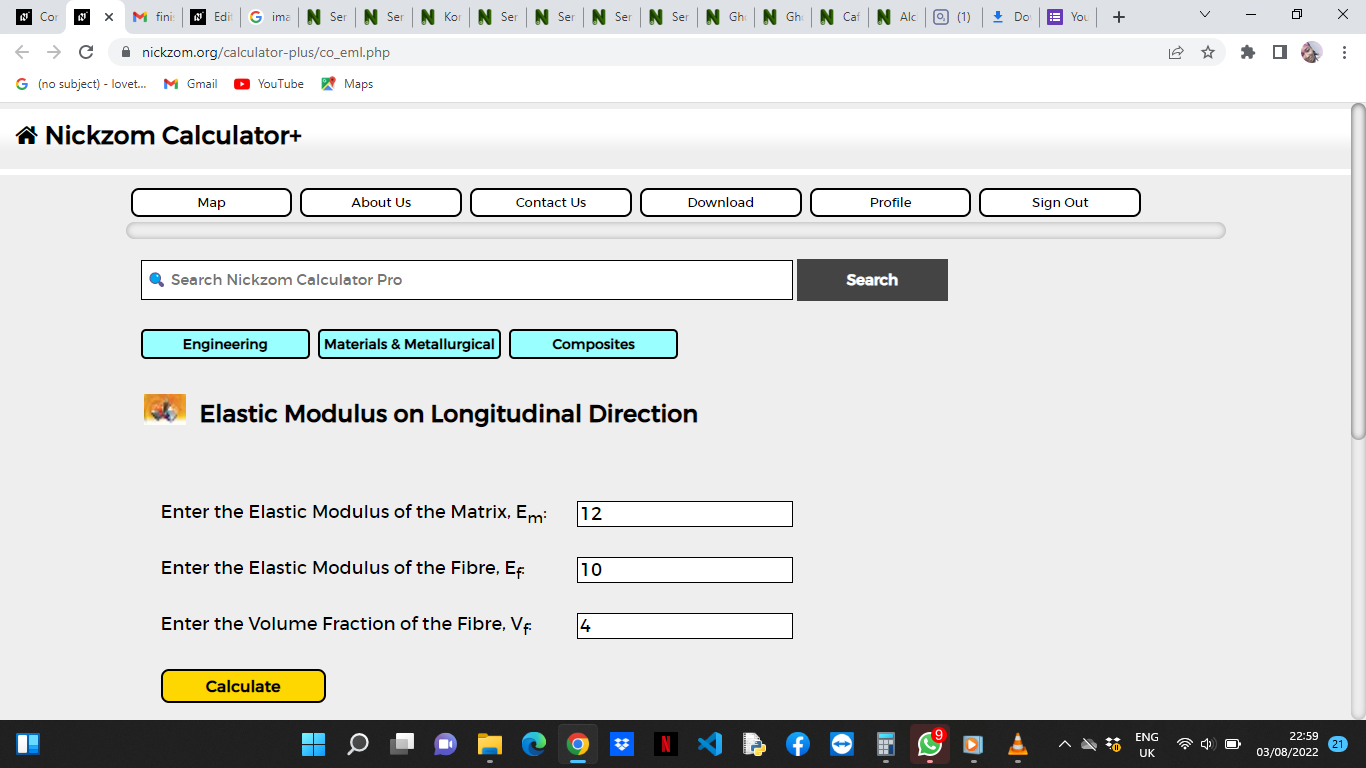Finally, Click on Calculate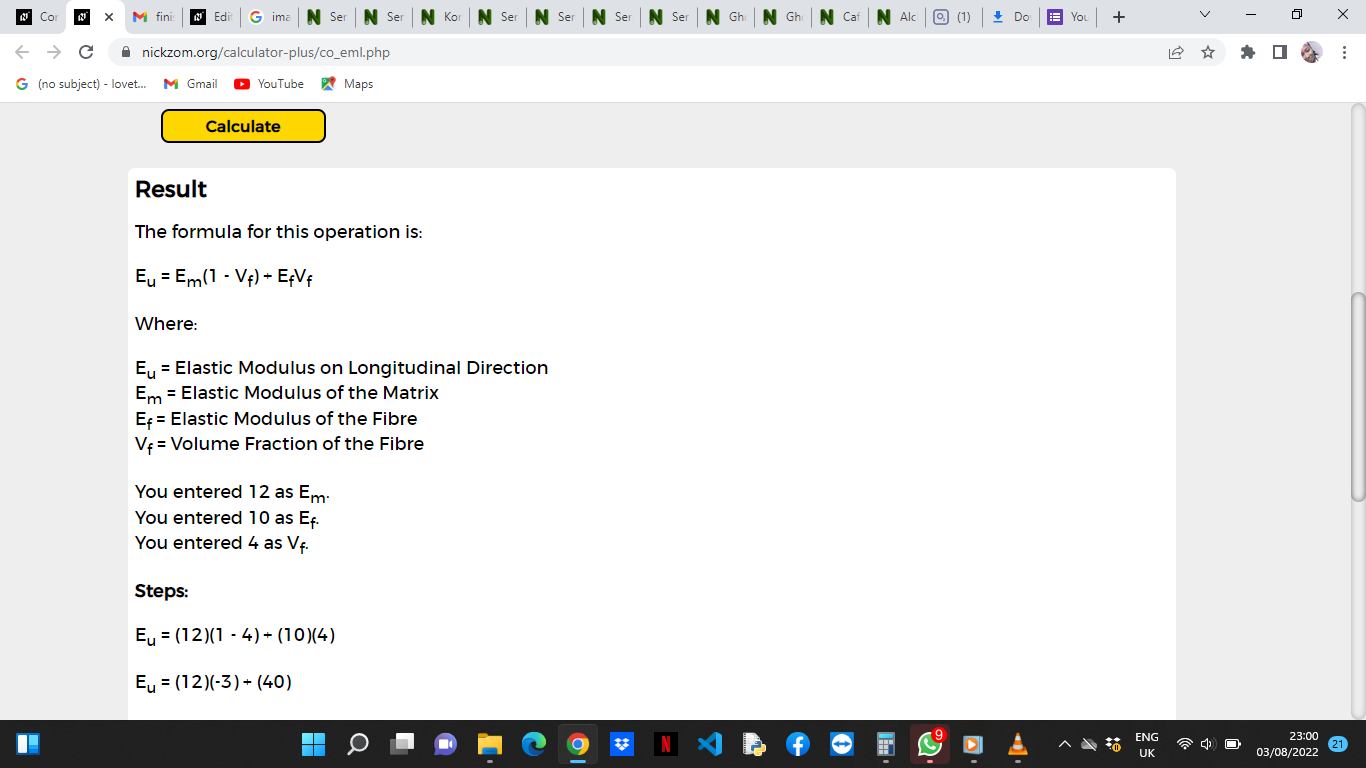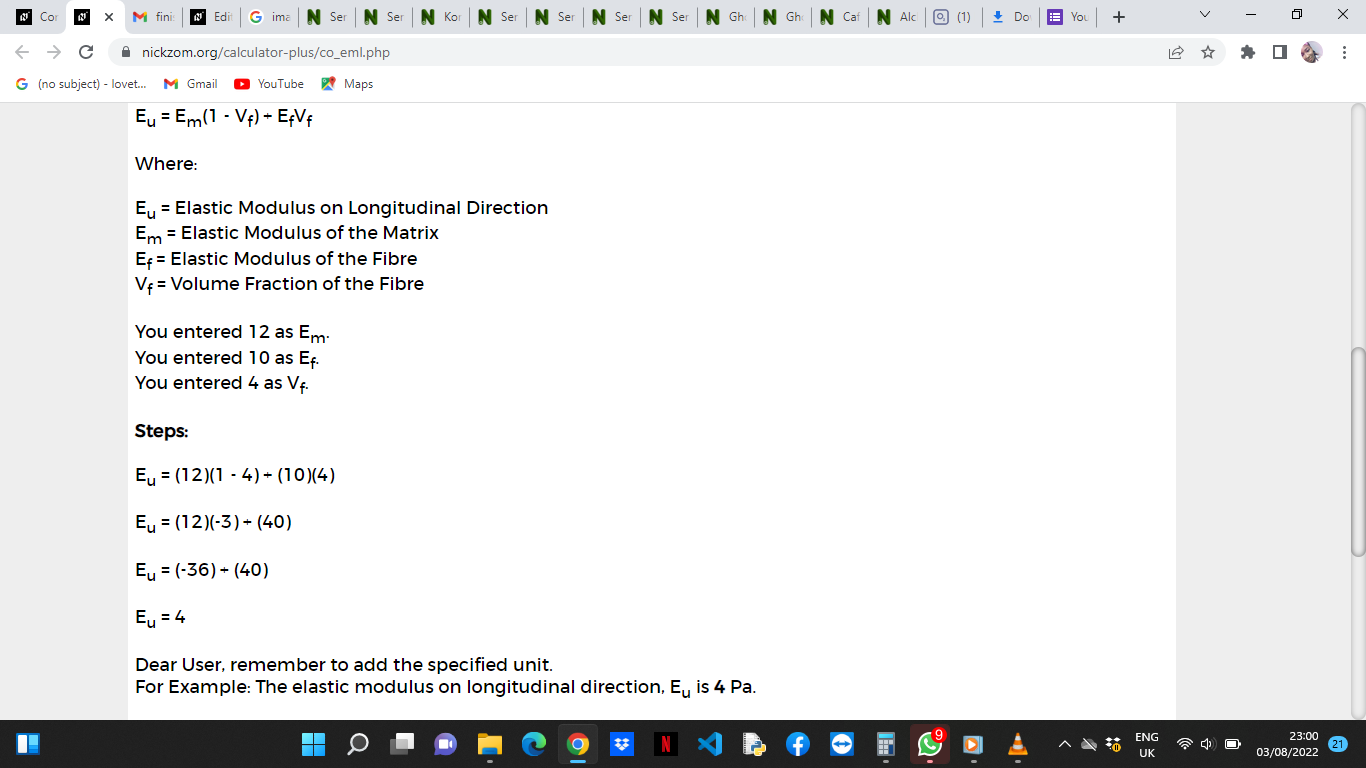As you can see from the screenshot above, Nickzom Calculator– The Calculator Encyclopedia solves for the elastic modulus on longitudinal directom and presents the formula, workings and steps too.